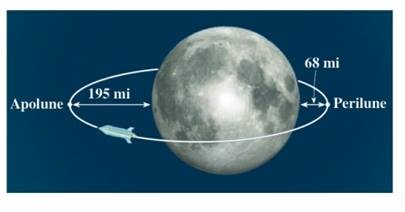Chapter 12.2, Problem 67E### Algebra and Trigonometry (MindTap ...

4th Edition
James Stewart + 2 others
ISBN: 9781305071742

#### Solutions

Chapter
Section### Algebra and Trigonometry (MindTap ...

4th Edition
James Stewart + 2 others
ISBN: 9781305071742
Textbook Problem

# APPLICATIONSLunar Orbit For an object in an elliptical orbit around the moon, the points in the orbit that are closest to and farthest from the center of the moon are called perilune and apolune, respectively. These are the vertices of the orbit. The Apollo 11 spacecraft was placed in a lunar orbit with perilune at 68 mi and apolune at 195 mi above the surface of the moon. Assuming that the moon is a sphere of radius 1075 mi, find an equation for the orbit of Apollo 11. (Place the coordinate axes so that the origin is at the center of the orbit and the foci are located on the x-axis).To determine

To Find:

The equation of orbit of Apollo 11.

Explanation

Given:

Figure (1)

From the figure, radius of moon (r) is 1075 mi, the distance between spacecraft and moon at perilune (p) is 68mi and distance between spacecraft and moon at apolune (m) is 195mi.

Approach:

Let the coordinates of spacecraft be (a,0) at perilune and (a,0) at apolune and let the moon be at one of the foci (c,0).

Equation of ellipse with foci at (c,0) and (c,0), major axis 2a and minor axis 2b is:

x2a2+y2b2=1 ……(1)

Eccentricity e for this ellipse is given by,

e=ca ……(2)

Calculation:

The value of a can be calculated as,

2a=p+m+2r=68+195+2×1075a=24132=1206.5

a2=1455642.251455642

The distance between spacecraft and moon at perilune is 68 which is equal to ac

### Still sussing out bartleby?

Check out a sample textbook solution.

See a sample solution

#### The Solution to Your Study Problems

Bartleby provides explanations to thousands of textbook problems written by our experts, many with advanced degrees!

Get Started